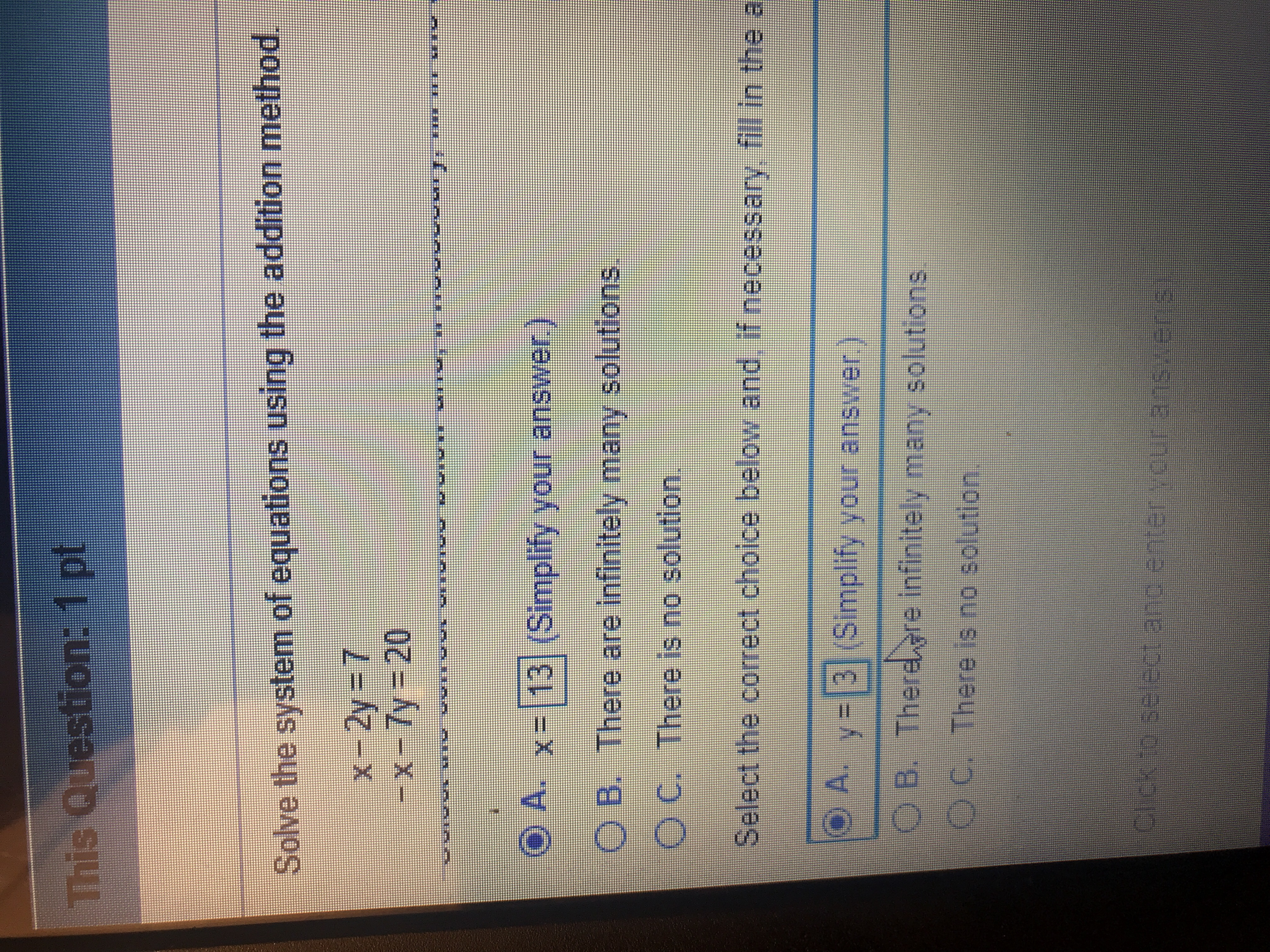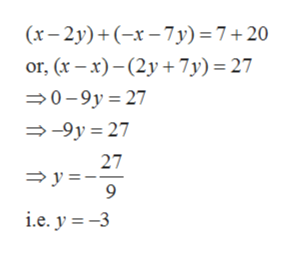# This Question: 1 ptSolve the system of equations using the addition methodX 2y 7-x-7y 20O AX- 13 (Simplify your answer,O B. There are infinitely many solutionsc. There is no solution,Select the correct choice below and, if necessary fill in the aA. y 3|(Simplilfy your answerDB. Therere infinitely many solutionsO c. There is no solutionChok to se act and enterOur 31516rsi

Question
3 viewshelp_outlineImage TranscriptioncloseThis Question: 1 pt Solve the system of equations using the addition method X 2y 7 -x-7y 20 O A X- 13 (Simplify your answer, O B. There are infinitely many solutions c. There is no solution, Select the correct choice below and, if necessary fill in the a A. y 3|(Simplilfy your answer DB. Therere infinitely many solutions O c. There is no solution Chok to se act and enterOur 31516rsi fullscreen
check_circle

Step 1

The given system of equation are

Step 2

To solve these equations using addition method, adding (1) and (2) giveshelp_outlineImage Transcriptionclose(x-2y)(x7y) = 7 + 20 or, (x-x)(2y7y) = 27 0-9y 27 -9y 27 27 y- i.e. y -3 fullscreen
Step 3

Plugging, y=-3 in...

### Want to see the full answer?

See Solution

#### Want to see this answer and more?

Solutions are written by subject experts who are available 24/7. Questions are typically answered within 1 hour.*

See Solution
*Response times may vary by subject and question.
Tagged in

### Equations and In-equations